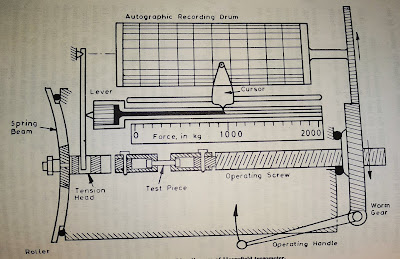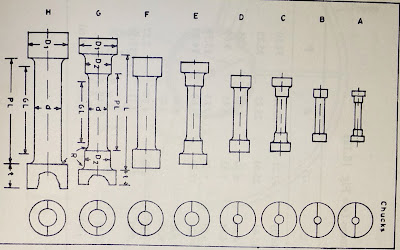# TENSILE TEST OF MATERIALS

## Tensile Test

Tensile test is one of the most widely performed tests. In this process we determined yield stress, upper and lower yield points, tensile strength, elongation and reduction in area.
● Universal tensile testing machine is frequently used for performing tensile test. The specimen is subjected to tensile load till fracture occurs. These results of tensile tests can be represented in the from of engineering or true stress-strain curve.

#### Yield Stress :Stress strain curve

The yield stress is defined as the stress at which plastic deformation of the tensile specimen takes place at a constant load. For example : carbon and low carbon steels in which elongation and extension have occurred.

### Tensile Strength :

● The tensile strength also known as ultimate strength which is denfind as the maximum stress withstand capacity of a material. It is obtained by dividing maximum load by original cross sectional area. Tensile strength value provides idea about hardness and fatigue strength of the material.

● When two samples of the same material with identical microstructure but with different composition are taken in to consideration, it observed that their fatigue strength and hardness bear directly relation to tensile strength.

Let's consider steels : The tensile strength in Newton per square (N/mm2) is related to its hardness in BHN like

Tensile strength : 3.242 × BHN for heat treated alloy steels.
Tensile strength : 3.396 × BHN for heat treated medium carbon steels.
Tensile strength : 3.551 × BHN for heat treated low carbon steels.

● Fatigue strength of most of the steel is of the 50% of tensile strength.

#### Elongation :

Elongation is generally given by change in length per unit length of the tensile specimen multiplied by 100. The change in length is always measured relative to some length marked on the tensile specimen prior to testing which is referred to as gauge length. The gauge length is always reported along with the reported value of percentage elongation.

Percentage elongation :     (Lf - Lo) × 100 / Lo
Where Lf : final length
Lo : initial length (gauge length)

Percentage reduction in area =   (Ao - Af) ×100 / Ao
Where Ao : original cross sectional area of the tensile specimen.
Af : final cross sectional area of the tensile specimen.Line diagram of hounsfield tensometer

For research work and in laboratories, Hounsfield tensometer is most commonly used. Load on tensile specimen in this tester a applied with the help of spring beams. The maximum load which can be applied on tensile specimen in a tensometer is 2000kg. Other loads available with tensometer are 1000kg, 500kg, 250kg, 125kg, 62.50kg, and 31.25kg. So a large number of materials can be tested on this machine. For reproducibility of results, samples of standard size should be tested.Standard tensile test specimens of hounsfield tensometer